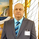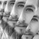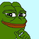Dilihat 1579 kali
1579
This is a study geared toward identifying price trends using Quadratic regression.

Quadratic regression is the process of finding the equation of a parabola that best fits the set of data being analyzed.

In this study, first a quadratic regression curve is calculated, then the slope of the curve is calculated and plotted.

Custom bar colors are included. The color scheme is based on whether the slope is positive or negative, and whether it is increasing or decreasing.Would variables x, t, and n take the place of the out of the function declared variables such as src, per and smper? Thanks.
Balassyracusepro
@syracusepro, my apologies on the late response. I've been working on a lot of projects lately.
To answer your question, QRS requires two inputs, x and t, which correspond to src and per in the script, respectively. smper is the sampling period for exponential smoothing of the QRS outside the function's definitions. n is the current bar number.
BalasGreat work. It’s great to see you publishing again. I’m looking forward to see what demons you release over the next while.
Balas